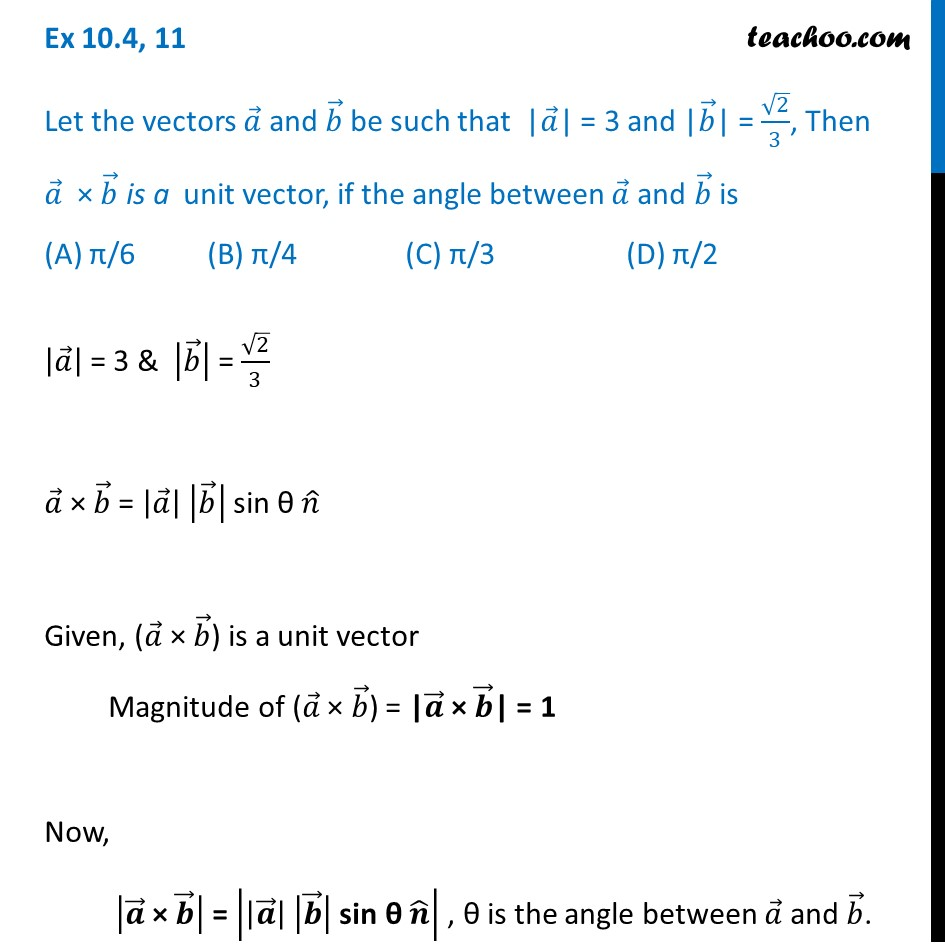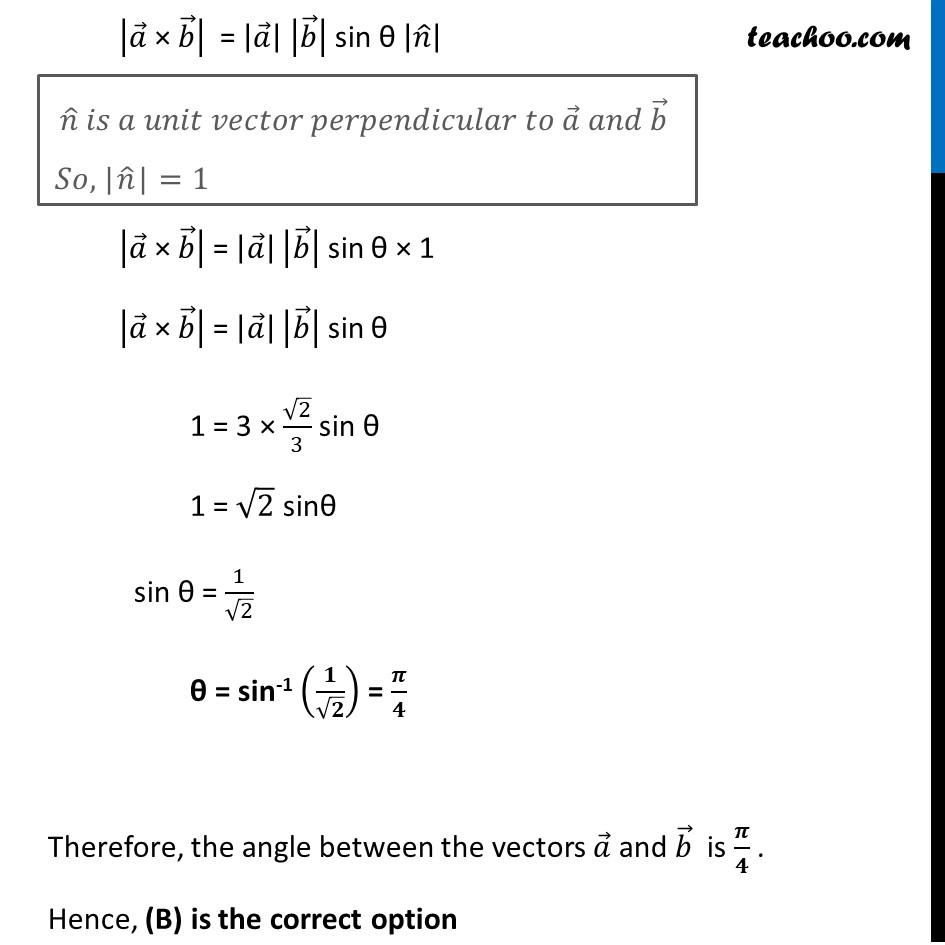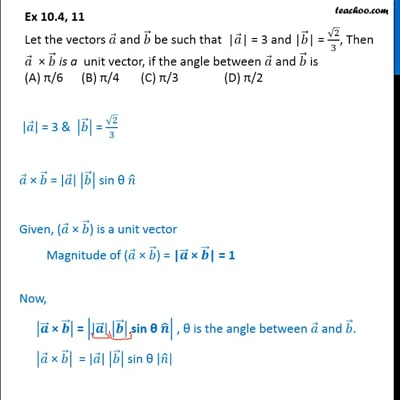Vector product - Defination

Chapter 10 Class 12 Vector Algebra
Concept wiseThis video is only available for Teachoo black users

Introducing your new favourite teacher - Teachoo Black, at only ₹83 per month

### Transcript

Ex 10.4, 11 Let the vectors 𝑎 ⃗ and 𝑏 ⃗ be such that |𝑎 ⃗| = 3 and |𝑏 ⃗| = √2/3, Then 𝑎 ⃗ × 𝑏 ⃗ is a unit vector, if the angle between 𝑎 ⃗ and 𝑏 ⃗ is (A) π/6 (B) π/4 (C) π/3 (D) π/2 |𝑎 ⃗ | = 3 & |𝑏 ⃗ | = √2/3 𝑎 ⃗ × 𝑏 ⃗ = |𝑎 ⃗ | |𝑏 ⃗ | sin θ 𝑛 ̂ Given, (𝑎 ⃗ × 𝑏 ⃗) is a unit vector Magnitude of (𝑎 ⃗ × 𝑏 ⃗) = |𝒂 ⃗ × 𝒃 ⃗| = 1 Now, |𝒂 ⃗" × " 𝒃 ⃗ | = |(|𝒂 ⃗ |" " |𝒃 ⃗ |" sin θ " 𝒏 ̂ )| , θ is the angle between 𝑎 ⃗ and 𝑏 ⃗. |𝑎 ⃗" × " 𝑏 ⃗ | = |𝑎 ⃗ | |𝑏 ⃗ | sin θ |𝑛 ̂ | |𝑎 ⃗" × " 𝑏 ⃗ | = |𝑎 ⃗ | |𝑏 ⃗ | sin θ × 1 |𝑎 ⃗" × " 𝑏 ⃗ | = |𝑎 ⃗ | |𝑏 ⃗ | sin θ 1 = 3 × √2/3 sin θ 1 = √2 sinθ sin θ = 1/√2 θ = sin-1 (𝟏/√𝟐) = 𝝅/𝟒 Therefore, the angle between the vectors 𝑎 ⃗ and 𝑏 ⃗ is 𝝅/𝟒 . Hence, (B) is the correct option 𝑛 ̂ 𝑖𝑠 𝑎 𝑢𝑛𝑖𝑡 𝑣𝑒𝑐𝑡𝑜𝑟 𝑝𝑒𝑟𝑝𝑒𝑛𝑑𝑖𝑐𝑢𝑙𝑎𝑟 𝑡𝑜 𝑎 ⃗ 𝑎𝑛𝑑 𝑏 ⃗ 𝑆𝑜,"|" 𝑛 ̂"|"=1